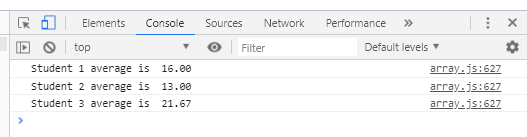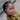# Jagged Array in JavaScript

Working with `jagged array` in most programming languages can be a bit clumsy , but the case is literally not the same for `JavaScript` because we easily get the length of the any row. You may be wondering what in the world is a `jagged array`, its fine, I presume this is your first time hearing it.

A `ragged array`, also known as a `jagged array`, is an array of arrays of which the member arrays can be of different sizes and producing rows of jagged edges when visualized as output. — Wikipedia

To give a clear understanding, lets imagine the measurement array where students have an unequal number of measurements recorded. We can still compute the correct average for each student without changing the program

``````var measurements = [[22, 10],[10, 13, 15, 14],[5, 27, 33]];
var total = 0;
var average = 0.0;
for (var row = 0; row < measurements.length; row++) {
for (var col = 0; col < measurements[row].length; col++) {
total += measurements[row][col];
}
average = total / measurements[row].length;
console.log(`Student \${parseInt(row+1)} average is  \${average.toFixed(2)}`);
total = 0;
average = 0.0;
}``````

observe that the first student only has two measurements, while the second student has four measurements, and the third student has three measurements respectively. Here is the output from the program:#JavaScript #arrayArticles written with 💛 by
Judith Oiku